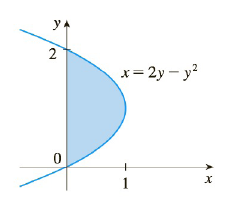Chapter 4.4, Problem 45E

Chapter
Section
Textbook Problem

# The area of the region that lies to the right of the y-axis and to the left of the parabola x = 2 y − y 2 (the shaded region in the figure) is given by the integral ∫ 0 2 ( 2 y − y 2 ) d y . (Turn your head clockwise and think of the region as lying below the curve x = 2 y − y 2 from y = 0 to y = 2 Find the area of the region.To determine

To find:

The area of the given region.

Explanation

1) Concept:

The fundamental theorem of calculus part 2: If  f  is continuous on a,b, then abfxdx=Fb-Fa,  where  F  is any antiderivative of f , that is, the function  F  such that F'=f .

2) Formula:

fx+gxdx=fxdx+gxdx

xndx=xn+1n+1+C

kdx=kx+C

3) Given:

The equation of the curve is f(y)=x=2y-y2

### Still sussing out bartleby?

Check out a sample textbook solution.

See a sample solution

#### The Solution to Your Study Problems

Bartleby provides explanations to thousands of textbook problems written by our experts, many with advanced degrees!

Get Started

#### Expand each expression in Exercises 122. (x+yxy)2

Finite Mathematics and Applied Calculus (MindTap Course List)

#### Differentiate. H(u)=(uu)(u+u)

Single Variable Calculus: Early Transcendentals, Volume I

#### Find each value of x. log7x=1

College Algebra (MindTap Course List)